# 2. Consider the following stress matrix:-r3x2GPa-12O33Find an expression for o33 such that the stress matrix satisfies the equations of equilibriumin the presence of body force vector b = -gez , where g is a constant.

Question
1 views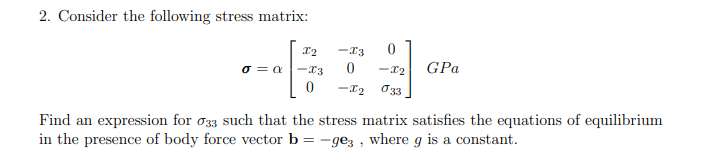help_outlineImage Transcriptionclose2. Consider the following stress matrix: -r3 x2 GPa -12 O33 Find an expression for o33 such that the stress matrix satisfies the equations of equilibrium in the presence of body force vector b = -gez , where g is a constant. fullscreen
check_circle

Step 1

The given stress matrix is,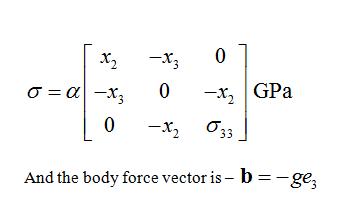Step 2

The given stress can be written as,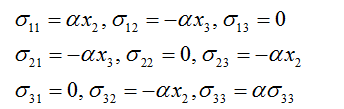Step 3

Writing the equilibrium equation for the matrix –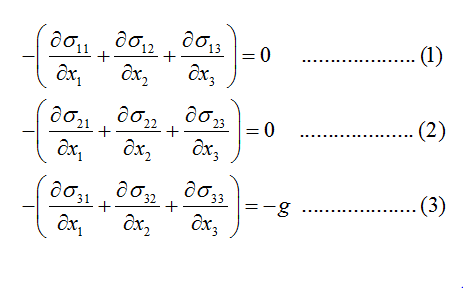From equation (3),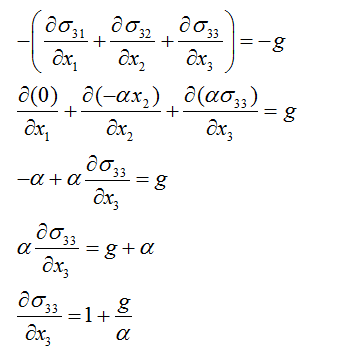...

### Want to see the full answer?

See Solution

#### Want to see this answer and more?

Solutions are written by subject experts who are available 24/7. Questions are typically answered within 1 hour.*

See Solution
*Response times may vary by subject and question.
Tagged in